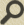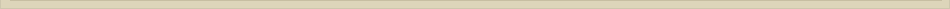# Lesson 2: Truth Tables and Equivalence

All course documents available here.

# Problems

• 1.1.1Which of the following are propositions?
(Solution, 99hertz )
• 1.1.2 Make truth tables for each of the following propositional forms.
(Solution, bspang)
• 1.1.3 If P, Q, and R are true while S and T are false, which of the following are true
(Solution, nikhilm92, sausagegobbler, jamesdthomson)
• 1.1.4 Which of the following pairs of propositional forms are equivalent?
(Solution, yottayottayottabyte, sausagegobbler)
• 1.1.5Use propositions A, B, and C to write the propositional form of each of the following. Decide which are true
(Solution, sausagegobbler, jamesdthomson )
• 1.1.6 P, Q, and R are propositional forms, and P is equivalent to Q, and Q is equivalent to R. Prove the following.
(Solution not available yet; Hint)
• 1.1.7 Let P be the sentence “Q is true” and Q be the sentence “P is false.” Is P a proposition? Explain.
(Solution, nikhilm92, jamesdthomson)
• 1.1.8 Determine whether each of the following is a tautology, a contradiction, or neither. Prove your answers
(Solution, Druzil)
• 1.1.9 Suppose A is a tautology and B is a contradiction. Are the following tautologies, contradictions, or neither?
(Solution, sausagegobbler, nikhilm92)
• 1.1.11 Restore parentheses to these abbreviated propositional forms.
(Solution,  mattde)